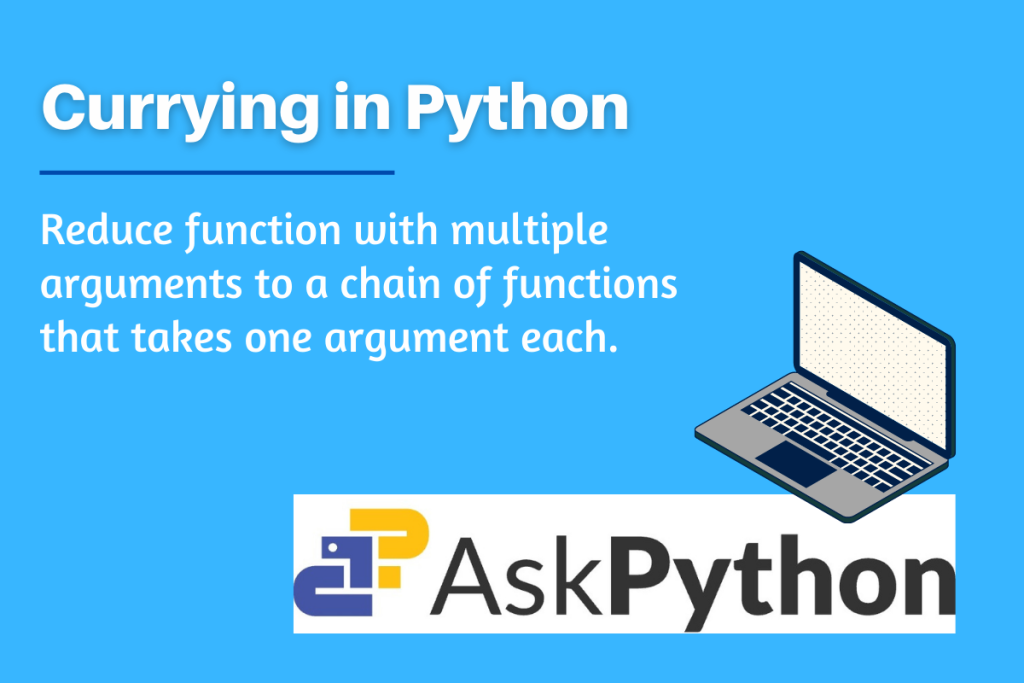# Currying in Python – A Beginner’s IntroductionIn this article, we will try to understand the concept “Curry, its advantages, and its implementation in python. Currying is actually named in honor of the mathematician and logician Haskell Curry. It is one of the functional design patterns. It is mainly used for problem-solving and programs designed on the concept of mathematical functions.

## What are Design Patterns?

Design Pattern provides a standard solution to a common or repeatedly occurring problem. The use of design patterns is very convenient and helps developers to increase the readability of the code they are working on.

## What is Currying?

Currying one such functional design pattern is primarily used to reduce function with multiple arguments to a chain of functions that takes one argument each.

For Example:

```function_mult(1,2,3) ---> function_mult(1)(2)(3)
```

Consider the function on the left side that performs multiplication and has three arguments 1, 2, and 3 and then produces output considering the values of all three arguments.

After performing Currying, the function is changed into a function with a single argument, this function takes the first argument (here 1) and returns a new function that takes the second argument (here 2), this function then again returns a new function that takes a third argument (here 3) and then produces the final output.

This is how currying changes one function with multiple arguments into a chain consisting of multiple functions of a single argument.

## How do we implement Currying in Python?

To understand the implementation of currying, let’s first define a function with multiple arguments.

Consider the following function that performs multiplication of the provided arguments.

```def mult(x, y, z):
return x * y * z

ans = mult(10, 20, 30)
print(ans)
```

Output:

6000

The initial step of currying is to bind the multiple arguments together. Consider the function has n arguments, and we need to bind all these arguments, for this we fix the function with the first argument and create a new function that takes (n – 1) arguments. Now we continue creating new functions until the number of arguments taken by the function is 1.

In Python, we use standard python function partial from functools

```from functools import partial

mult_10 = partial(mult, 10)
mult_10_20 = partial(mult_10, 20)
print(mult_10_20(30))
```

Output:

6000

## Currying using Decorator

Currying can be implemented much more efficiently by using the decorator. A decorator wraps code or functionality around a function in order to enhance what the function does. For that, we use different standard python functions. (To learn more about decorators click here)

Firstly we use signature, it helps to keep a count on the number of arguments passed to the function.

Partial functions here help in deriving a function with n parameters to a function with fewer parameters

```from inspect import signature
def curry(func):

def inner(arg):

#checking if the function has one argument,
#then return function as it is
if len(signature(func).parameters) == 1:
return func(arg)

return curry(partial(func, arg))

return inner

#using cuury function on the previous example function
@curry
def mult(x, y, z):
return x * y * z

print(mult(10)(20)(30))
```

Output:

6000

## Get started currying

Curry is a functional design pattern. The primary objective of using curry is to reduce a given function into a sequence of binding functions, this makes the jobs of developers more convenient and makes the solution more readable. In this article, we have tried to understand the working and implementation of Curry in Python language.